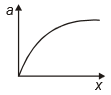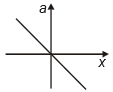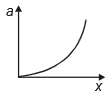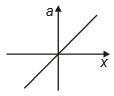You’ve reached the end of your free Videos limit.
#37-Ball in Neck of Air Chamber
(Physics) > Oscillations
Unable to watch the video, please try another server
Related Practice Questions :

The variation of acceleration of a particle executing SHM with displacement x is

1.2.3.4.High Yielding Test Series + Question Bank - NEET 2020

Difficulty Level:

A particle is subjected to two simple harmonic motions in the same direction having equal amplitudes and equal frequency. If the resulting amplitude is equal to the amplitude of individual motions, the phase difference between them is:

1.  $\frac{\mathrm{\pi }}{3}$

2.  $\frac{2\mathrm{\pi }}{3}$

3.  $\frac{\mathrm{\pi }}{6}$

4.  $\frac{\mathrm{\pi }}{2}$

High Yielding Test Series + Question Bank - NEET 2020

Difficulty Level:

The motion of a particle varies with time according to the relation . Then

1.  The motion is oscillatory but not SHM

2.  The motion is SHM with amplitude $a\sqrt{2}$

3.  The motion is SHM with amplitude $\sqrt{2}$

4.  The motion is SHM with amplitude a

High Yielding Test Series + Question Bank - NEET 2020

Difficulty Level:

If a particle is executing SHM, with an amplitude A, the distance moved and the displacement of the body in a time equal to its period are

1.  2A, A

2.  4A, 0

3.  A, A

4.  0, 2A

High Yielding Test Series + Question Bank - NEET 2020

Difficulty Level:

A particle undergoes SHM with a time period of 2 seconds. In how much time will it travel from its mean position to a displacement equal to half of its amplitude?

(1) $\frac{1}{2}s$

(2) $\frac{1}{6}s$

(3) $\frac{1}{4}s$

(4) $\frac{1}{3}s$